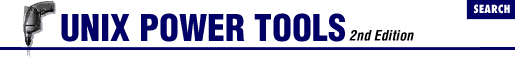home | O'Reilly's CD bookshelfs | FreeBSD | Linux | Cisco | Cisco Exam

#Chapter 9Saving Time on the Command Line## 9.6 String Editing (Colon) Operators

When the C shell and bash do history substitutions (11.7 ) they can also edit the substitution. The C shell - but not bash  - can also edit variable substitutions (6.8 ) . For instance, in the first example below, when `!\$` contains `/a/b/c` , adding the "head" operator `:h` will give just the head of the pathname, `/a/b` .

For a complete but very terse list of these operators, see the csh manual page. We hope the examples below will help you understand these useful operators.

• ```:h ``` gives the head of a pathname (14.2 ) , as follows:

```% ```echo /a/b/c
```

/a/b/c
% ```echo !\$:h
```

echo /a/b
/a/b```

That took off the filename and left the header. This also could be used with C shell variables (47.5 ) as:

```% ```set x = /a/b/c
```

% ```echo \$x
```

/a/b/c
% ```echo \$x:h
```

/a/b```

• ```:r ``` returns the root of a filename:

```% ```echo xyz.c abc.c
```

xyz.c abc.c
% ```echo !\$:r
```

echo abc
abc```

The `:r` removed the `.c` from the last argument, leaving the root name. This could also be used in C shell variable names:

```% ```set x = abc.c
```

% ```echo \$x:r
```

abc```

• ```:g ``` For more than one name, you can add the g operator to make the operation global. For example:

```(...) ``` ```% ```set x = (a.a b.b c.c) ``` % ```echo \$x:gr ``` a b c```

The `:gr` operator stripped off all dot (`.` ) suffixes. By the way, this use of g does not work with the history commands.

This is the C shell's answer to the basename (45.18 ) command.

• ```:e ``` returns the extension (the part of the name after a dot). Using csh variables:

```% ```set x=(abc.c)
```

% ```echo \$x:e
```

c```

No luck using that within history, either.

• ```:t ``` gives the tail of a pathname - the actual filename without the path:

```% ```echo /a/b/c
```

/a/b/c
% ```echo !\$:t
```

c```

With csh variables:

```% ```set x=(/a/b/c)
```

% ```echo \$x:t
```

c```

And with multiple pathnames, you can do it globally with:

```% ```set x=(/a/b/c /d/e/f /g/h/i)
```

% ```echo \$x:gt
```

c f i```

While the corresponding heads would be:

```% ```set x=(/a/b/c /d/e/f /g/h/i)
```

% ```echo \$x:gh
```

/a/b /d/e /g/h```

• ```:p ``` prints the command, but does not execute it (11.10 ) :

```% ```echo *
```

fn1 fn2 fn3
% ```!:p
```

echo fn1 fn2 fn3```

• ```:q ``` prevents further filename expansion, or prints the command as is:

```% ```echo *
```

fn1 fn2 fn3
% ```!:q
```

echo *
*```

The first command echoed the files in the directory, and when the `:q` was applied, it echoed only the special character.

• ```:x ``` is like `:q` , but it breaks the line into words. That is, when using `:q` , it is all one word, while `:x` will break it up into multiple words. [`:q` and `:x` are more often used with C shell arrays (47.5 ) . -JP  ]

- DR9.5 Build Strings with { }9.7 String Editing in ksh and bash